DLPinchableView is Custom Library for iOS written in Objective-C. Pinch, Rotate, and Pan your Image View. I really like UI/UX, this custom view was inspired from Instagram application, then i decided to challenge myself to create  my own version of it. Checkout my github project to see this in action!

## How It works?

Basically is quite simple, i created class called DLPinchableView extend from UIImageView,  then adding this of

Everything is triggered by UIPinchGestureRecognizer. Firstly when the view is pinched, i save origin location CGRect, then i hide the original view, and add DLParentPinchableView *duplicate the original view and adding black fade background.

Thanks to Core Graphics Framework everything become so easy by applying CGAffineTransform from the Gesture Events

## Implementation

Simple AF

• Using Interface Builder ( .xib or .storyboard )Drag UIImageView to your Interface Builder then change Class Name to DLPinchableView.
Magic!

• Programmatically
``````DLPinchableView *imgV = [[DLPinchableView alloc]initWithFrame:CGRectMake(0, 0, 300, 300)];
imgV.image = [UIImage imageNamed:@"ImgCat1"];

## The Math Behind the Matrices

The only Quartz 2D function for which you need an understanding of matrix math is the function CGAffineTransformMake, which makes an affine transform from the six critical entries in a 3 x 3 matrix. Even if you never plan to construct an affine transformation matrix from scratch, you might find the math behind the transform functions interesting. If not, you can skip the rest of this chapter.

The six critical values of a 3 x 3 transformation matrix —a, b, c, d, tx and ty— are shown in the following matrix: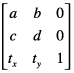Note: The rightmost column of the matrix always contains the constant values 0, 0, 1. Mathematically, this third column is required to allow concatenation, which is explained later in this section. It appears in this section for the sake of mathematical correctness only.

Given the 3 x 3 transformation matrix described above, Quartz uses this equation to transform a point (x, y) into a resultant point (x’, y’):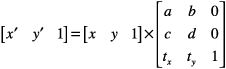The result is in a different coordinate system, the one transformed by the variable values in the transformation matrix. The following equations are the definition of the previous matrix transform: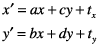The following matrix is the identity matrix. It performs no translation, scaling, or rotation. Multiplying this matrix by the input coordinates always returns the input coordinates.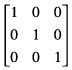Using the formulas discussed earlier, you can see that this matrix would generate a new point (x’, y’) that is the same as the old point (x, y):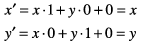This matrix describes a translation operation: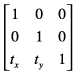These are the resulting equations that Quartz uses to apply the translation: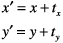This matrix describes a scaling operation on a point (x, y):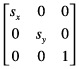These are the resulting equations that Quartz uses to scale the coordinates: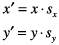This matrix describes a rotation operation, rotating the point (x, y) counterclockwise by an angle a: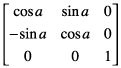These are the resulting equations that Quartz uses to apply the rotation: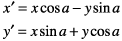This equation concatenates a rotation operation with a translation operation: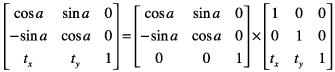These are the resulting equations that Quartz uses to apply the transform: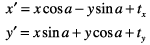Note that the order in which you concatenate matrices is important—matrix multiplication is not commutative. That is, the result of multiplying matrix A by matrix B does not necessarily equal the result of multiplying matrix B by matrix A.
As previously mentioned, concatenation is the reason the affine transformation matrix contains a third column with the constant values 0, 0, 1. To multiply one matrix against another matrix, the number of columns of one matrix must match the number of rows of the other. This means that a 2 x 3 matrix cannot be multiplied against a 2 x 3 matrix. Thus we need the extra column containing the constant values.
An inversion operation produces original coordinates from transformed ones. Given the coordinates (x, y), which have been transformed by a given matrix A to new coordinates (x’, y’), transforming the coordinates (x’, y’) by the inverse of matrix A produces the original coordinates (x, y). When a matrix is multiplied by its inverse, the result is the identity matrix.